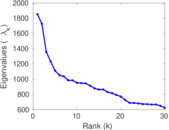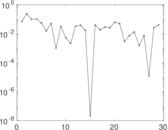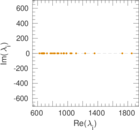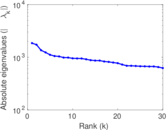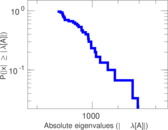This network consists of the wikilinks of the Wikipedia in the Serbo-Croatian language (sh). Nodes are Wikipedia articles, and directed edges are wikilinks, i.e., hyperlinks within one wiki. In the wiki source, these are indicated with [[double brackets]]. Only pages in the article namespace are included.

 Code `Wsh` Internal name `wikipedia_link_sh` Name Wikipedia links (sh) Data source http://dumps.wikimedia.org/ AvailabilityDataset is available for download Consistency checkDataset passed all tests Category Hyperlink network Node meaning Article Edge meaning Wikilink Network format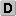Unipartite, directed Edge typeUnweighted, no multiple edges ReciprocalContains reciprocal edges Directed cyclesContains directed cycles Loops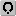Contains loops

## Statistics

 Size n = 3,924,218 Volume m = 106,265,500 Loop count l = 373 Wedge count s = 680,297,499,272 Claw count z = 17,914,530,919,777,664 Cross count x = 5.377 52 × 1020 Triangle count t = 14,933,040,455 Maximum degree dmax = 254,555 Maximum outdegree d+max = 6,003 Maximum indegree d−max = 254,554 Average degree d = 54.158 8 Size of LCC N = 3,924,147 Size of LSCC Ns = 460,773 Relative size of LSCC Nrs = 0.117 418 Diameter δ = 9 50-Percentile effective diameter δ0.5 = 3.474 67 90-Percentile effective diameter δ0.9 = 3.972 09 Median distance δM = 4 Mean distance δm = 3.956 16 Gini coefficient G = 0.947 468 Balanced inequality ratio P = 0.056 637 6 Outdegree balanced inequality ratio P+ = 0.068 129 1 Indegree balanced inequality ratio P− = 0.167 909 Relative edge distribution entropy Her = 0.792 833 Clustering coefficient c = 0.065 852 3 Directed clustering coefficient c± = 0.937 391 Operator 2-norm ν = 2,853.22 Reciprocity y = 0.561 642 Non-bipartivity bA = 0.447 929 Normalized non-bipartivity bN = 0.036 310 7

## Plots

### Degree distribution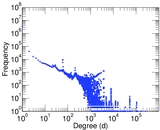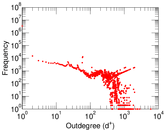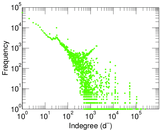### Cumulative degree distribution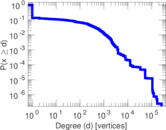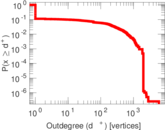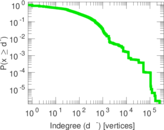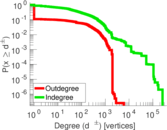### Hop distribution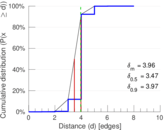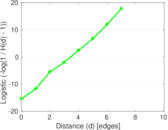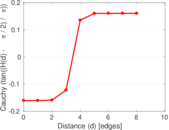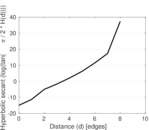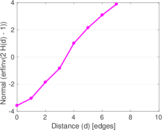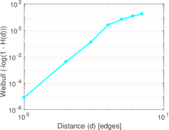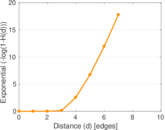### In/outdegree scatter plot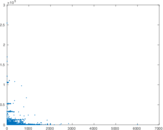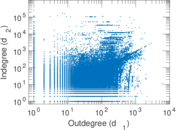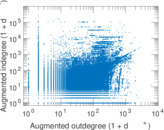### Matrix decompositions plots